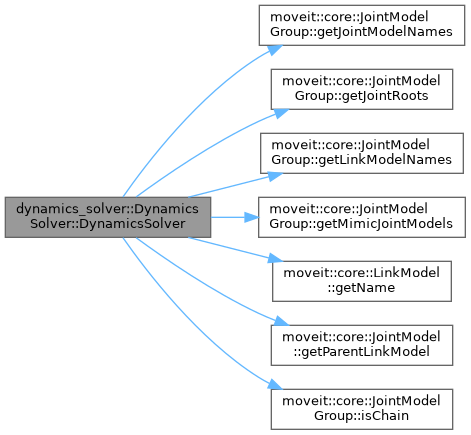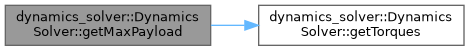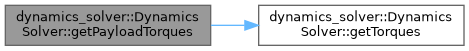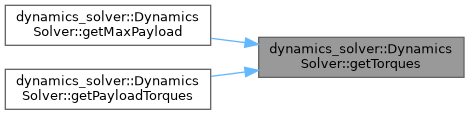moveit2 The MoveIt Motion Planning Framework for ROS 2.
dynamics_solver::DynamicsSolver Class Reference

`#include <dynamics_solver.h>`

## Public Member Functions

DynamicsSolver (const moveit::core::RobotModelConstPtr &robot_model, const std::string &group_name, const geometry_msgs::msg::Vector3 &gravity_vector)
Initialize the dynamics solver. More...

bool getTorques (const std::vector< double > &joint_angles, const std::vector< double > &joint_velocities, const std::vector< double > &joint_accelerations, const std::vector< geometry_msgs::msg::Wrench > &wrenches, std::vector< double > &torques) const
Get the torques (the order of all input and output is the same as the order of joints for this group in the RobotModel) More...

bool getMaxPayload (const std::vector< double > &joint_angles, double &payload, unsigned int &joint_saturated) const
Get the maximum payload for this group (in kg). Payload is the weight that this group can hold when the weight is attached to the origin of the last link of this group. (The order of joint_angles vector is the same as the order of joints for this group in the RobotModel) More...

bool getPayloadTorques (const std::vector< double > &joint_angles, double payload, std::vector< double > &joint_torques) const
Get torques corresponding to a particular payload value. Payload is the weight that this group can hold when the weight is attached to the origin of the last link of this group. More...

const std::vector< double > & getMaxTorques () const
Get maximum torques for this group. More...

const moveit::core::RobotModelConstPtr & getRobotModel () const
Get the kinematic model. More...

const moveit::core::JointModelGroupgetGroup () const

## Detailed Description

This solver currently computes the required torques given a joint configuration, velocities, accelerations and external wrenches acting on the links of a robot

Definition at line 58 of file dynamics_solver.h.

## ◆ DynamicsSolver()

 dynamics_solver::DynamicsSolver::DynamicsSolver ( const moveit::core::RobotModelConstPtr & robot_model, const std::string & group_name, const geometry_msgs::msg::Vector3 & gravity_vector )

Initialize the dynamics solver.

Parameters
 urdf_model The urdf model for the robot srdf_model The srdf model for the robot group_name The name of the group to compute stuff for
Returns
False if initialization failed

Definition at line 68 of file dynamics_solver.cpp.

Here is the call graph for this function:## ◆ getGroup()

 const moveit::core::JointModelGroup* dynamics_solver::DynamicsSolver::getGroup ( ) const
inline

Definition at line 131 of file dynamics_solver.h.

 bool dynamics_solver::DynamicsSolver::getMaxPayload ( const std::vector< double > & joint_angles, double & payload, unsigned int & joint_saturated ) const

Get the maximum payload for this group (in kg). Payload is the weight that this group can hold when the weight is attached to the origin of the last link of this group. (The order of joint_angles vector is the same as the order of joints for this group in the RobotModel)

Parameters
 joint_angles The joint angles (desired joint configuration) this must have size = number of joints in the group payload The computed maximum payload joint_saturated The first saturated joint and the maximum payload
Returns
False if the input set of joint angles is of the wrong size

Definition at line 218 of file dynamics_solver.cpp.

Here is the call graph for this function:## ◆ getMaxTorques()

 const std::vector< double > & dynamics_solver::DynamicsSolver::getMaxTorques ( ) const

Get maximum torques for this group.

Returns
Vector of max torques

Definition at line 319 of file dynamics_solver.cpp.

 bool dynamics_solver::DynamicsSolver::getPayloadTorques ( const std::vector< double > & joint_angles, double payload, std::vector< double > & joint_torques ) const

Get torques corresponding to a particular payload value. Payload is the weight that this group can hold when the weight is attached to the origin of the last link of this group.

Parameters
 joint_angles The joint angles (desired joint configuration) this must have size = number of joints in the group payload The payload for which to compute torques (in kg) joint_torques The resulting joint torques
Returns
False if the input vectors are of the wrong size

Definition at line 283 of file dynamics_solver.cpp.

Here is the call graph for this function:## ◆ getRobotModel()

 const moveit::core::RobotModelConstPtr& dynamics_solver::DynamicsSolver::getRobotModel ( ) const
inline

Get the kinematic model.

Returns
kinematic model

Definition at line 126 of file dynamics_solver.h.

## ◆ getTorques()

 bool dynamics_solver::DynamicsSolver::getTorques ( const std::vector< double > & joint_angles, const std::vector< double > & joint_velocities, const std::vector< double > & joint_accelerations, const std::vector< geometry_msgs::msg::Wrench > & wrenches, std::vector< double > & torques ) const

Get the torques (the order of all input and output is the same as the order of joints for this group in the RobotModel)

Parameters
 joint_angles The joint angles (desired joint configuration) this must have size = number of joints in the group joint_velocities The desired joint velocities this must have size = number of joints in the group joint_accelerations The desired joint accelerations this must have size = number of joints in the group wrenches External wrenches acting on the links of the robot this must have size = number of links in the group torques Computed set of torques are filled in here this must have size = number of joints in the group
Returns
False if any of the input vectors are of the wrong size

Definition at line 147 of file dynamics_solver.cpp.

Here is the caller graph for this function:The documentation for this class was generated from the following files: